DAY 16
1
Software Development

[2020鐵人賽Day16]糊裡糊塗Python就上手-Python Excel 操縱套餐(使用openpyxl)

What is Excel?

Excel 試算表亦稱作活頁簿(workbook)，而活頁簿我們會存在 .xlsx 的副檔名檔案中。

安裝 openpyxl 模組

Install module openpyxl

可在 Anaconda 裡查看有無安裝此模組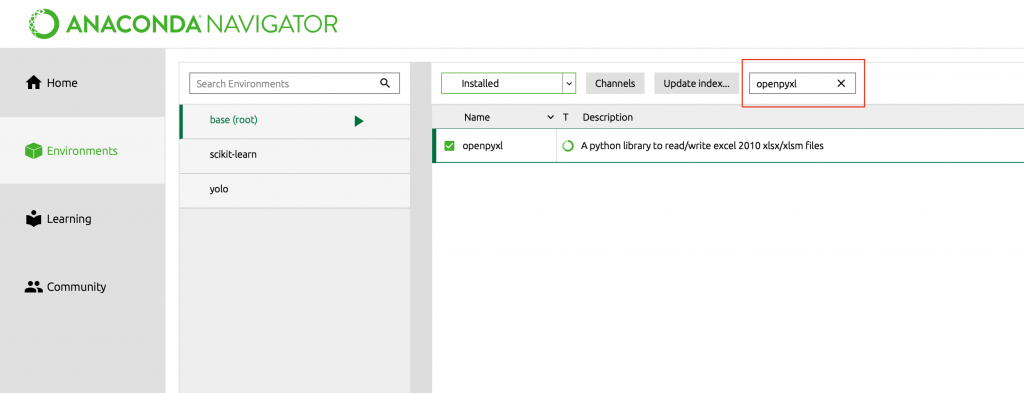另可在 Termianl 視窗中，利用以下的指令來進行openpyxl套件的安裝
pip install openpyxl

import openpyxl

實際體驗

新建一份Excel，並新增資料內容

使用openpyxl 內 Workbook 方法建立一個新的工作簿
sheet = workbook.worksheets
取得第一個工作表
sheet = workbook.worksheets
設定 sheet 工作表 A1 儲存格內容為 "Hello Python, Hello Excel."
sheet['A1'] = 'Hello Python, Hello Excel.'
儲存檔案
workbook.save('test.xlsx')
完整代碼
import openpyxl

# 利用 Workbook 建立一個新的工作簿
workbook = openpyxl.Workbook()

# 取得第一個工作表
sheet = workbook.worksheets

# 設定 sheet 工作表 A1 儲存格內容為 "Hello Python, Hello Excel."
sheet['A1'] = 'Hello Python, Hello Excel.'

# 儲存檔案
workbook.save('test.xlsx')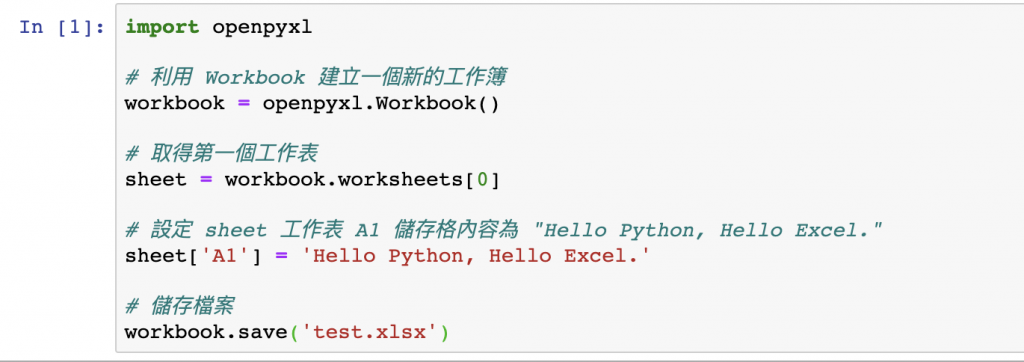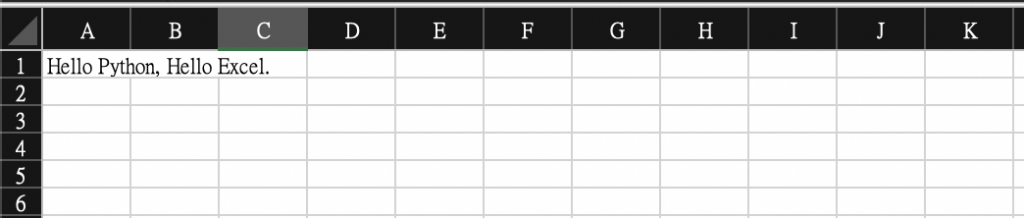讀取剛剛建立的Excel，並修改儲存格A1內容

其實跟上述做法雷同，將使用openpyxl 內 load_workbook 方法，讀取 Excel 檔案
import openpyxl

# 取得第一個工作表
sheet = workbook.worksheets

# 設定 sheet 工作表 A2 儲存格內容為 "Test Excel"
sheet['A2'] = 'Test Excel.'

# 儲存檔案
workbook.save('test.xlsx')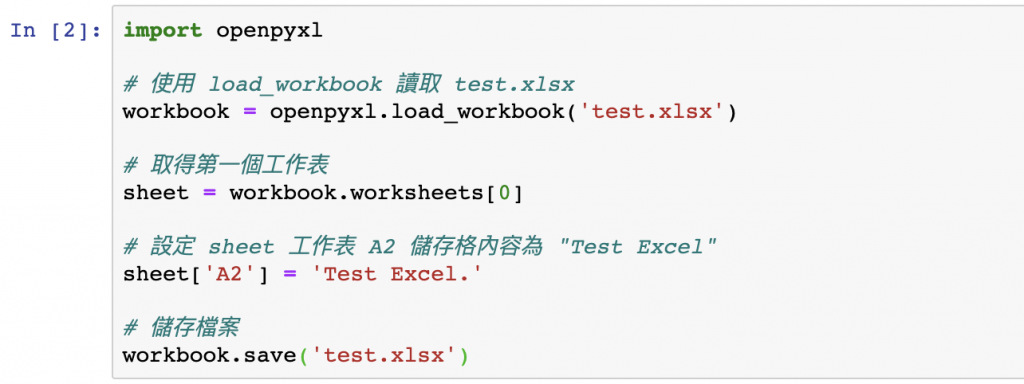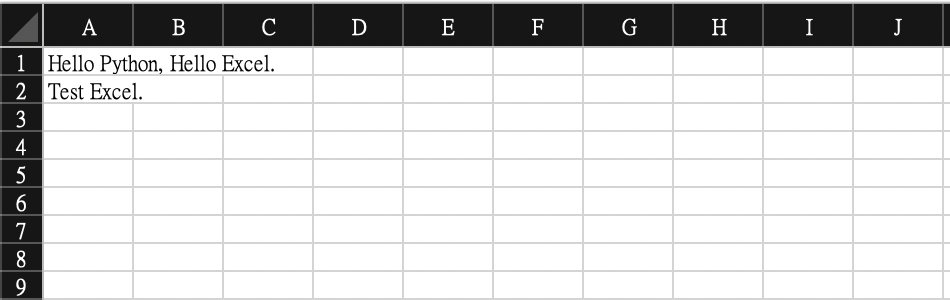顯示出剛修正後的「test.xlsx」內容的row及column總數，並把內容顯示出來

max_row:可取出row總數
max_column:可取出column總數

import openpyxl

# 取得第一個工作表
sheet = workbook.worksheets

# 顯示 row總數 及 column總數
print('row總數:', sheet.max_row)
print('column總數:', sheet.max_column)

# 顯示 cell 資料
for i in range(1, sheet.max_row+1):
for j in range(1, sheet.max_column+1):
print(sheet.cell(row = i, column = j).value)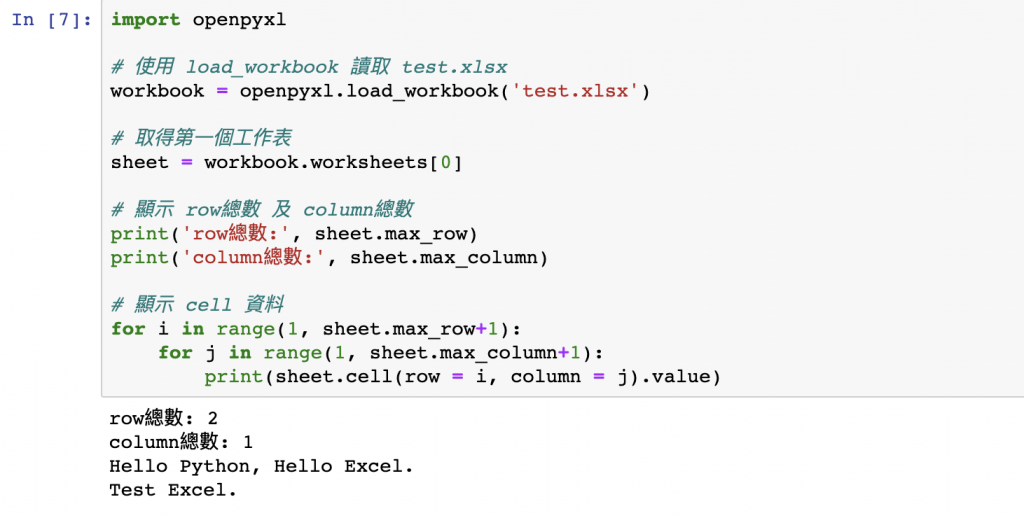結論

Excel 檔案其實也是挺常遇到的資料儲存格式，若能熟能生巧，相信日後要產出資料檔案，一定能事半功倍。